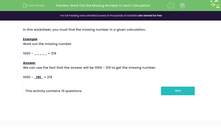# Work Out the Missing Number in Calculations

In this worksheet, students will find the missing number in a given calculation. Students could attempt the calculations in their heads first.Key stage:  KS 3

Curriculum topic:   Number

Curriculum subtopic:   Use Four Operations for All Numbers

Popular topics:   Numbers worksheets

Difficulty level:#### Worksheet Overview

In this activity, we will find the missing number in a given calculation.

Example

Work out the missing number:

1000 - _____ = 219

We can use the fact that 1000 - 219 will give us our missing number.

1000 - 219 = 781

1000 -   781   = 219

We will need to think about doing the inverse of calculations to solve many of these questions.

Let's get started!

### What is EdPlace?

We're your National Curriculum aligned online education content provider helping each child succeed in English, maths and science from year 1 to GCSE. With an EdPlace account you’ll be able to track and measure progress, helping each child achieve their best. We build confidence and attainment by personalising each child’s learning at a level that suits them.

Get started